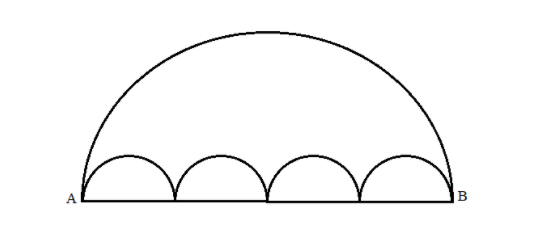Courses
Courses for Kids
Free study material
Offline Centres
MoreLast updated date: 10th Dec 2023
Total views: 280.8k
Views today: 6.80k

# All the arcs in the following diagram are semi-circles. This diagram shows two paths connecting A to B. Path $1$ is the single large semi-circle and path $2$ consists of the chain of small semi-circles. ThenA. Path $1$ is longer than path $2$ B. Path $1$ is of the same length as path $2$ C. Path $1$ is shorter than path $2$ D. Path $1$ is of the same length as path $2$ , only if the number of semi-circles is not more than $4$ .Verified
280.8k+ views
Hint: Some of the thing we need to know before solving this question:
Half of the circle is known as a semi-circle. Therefore, the area of the semi-circle will be half of the area of the circle.
Area of a circle is $\pi {r^2}$ then, area of a semicircle is $\dfrac{{\pi {r^2}}}{2}$ where $r$ is the radius of circle or radius of semi-circle. Perimeter of a semicircle is given by, $\pi r + 2r$ where $r$ is the radius of the semi-circle.

The given diagram consists of one large semi-circle and four small semi-circles. Thus, we have two paths to reach B from A.
It is given that the path $1$ from A to B is the large semi-circle that is the arc length of that semi-circle. That is the perimeter of a semi-circle minus its diameter, since this path doesn’t include the diameter. And it is given by $\pi r + 2r - d$ where $r$ is the radius of the semi-circle and $d$ is the diameter of the semi-circle.
We know that diameter is nothing but twice the radius that is, $d = 2r$ . Thus, we get, $\pi r + 2r + 2r$ .
On simplifying this we get, $\pi r$ . Thus, Path $1 =$ $\pi r$ .
Let’s calculate the path $2$ . It is given that path $2$ is the way along the small four semi-circles. That is nothing but the perimeter of those four semicircles minus their diameters, since the path doesn’t cover its diameters. Since all four semi-circles are equal, we get, $4 \times (\pi r)$ . Thus, Path $2 =$ $4\pi r$ .
On comparing both parts we get, Path $1 <$ Path $2$ . Since, $\pi r < 4\pi$ is obvious.
Therefore, Path $1$ is smaller than Path $2$ .
Let us see the options, option (a) Path $1$ is longer than path $2$ this cannot be a correct answer since Path $1$ is smaller than Path $2$ .
Option (b) Path $1$ is of the same length as path $2$ , also this cannot be the correct answer since Path $1$ is smaller than Path $2$ .
Option (c) Path $1$ is shorter than path $2$ , this is the correct answer since Path $1$ is smaller than Path $2$ .
Option (d) Path $1$ is of the same length as path $2$ , only if the number of semi-circles is not more than $4$ , this cannot be the correct answer since Path $1$ is smaller than Path $2$ .

So, the correct answer is “Option C”.

Note: Since the path is from A to B, we cannot walk through the diameter of the semicircle clearly, they said that we need to walk through the arc. Thus, the path is the perimeter of the semi-circle except the diameter thus we neglected the diameter from the formula. Same idea is applied for those four semicircles.